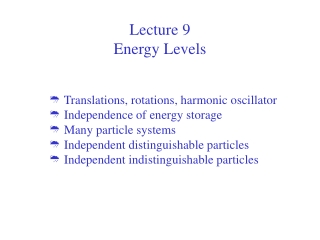Download PresentationLecture 9 Energy Levels

# Lecture 9 Energy Levels

Download Presentation## Lecture 9 Energy Levels

- - - - - - - - - - - - - - - - - - - - - - - - - - - E N D - - - - - - - - - - - - - - - - - - - - - - - - - - -
##### Presentation Transcript

1. Lecture 9 Energy Levels • Translations, rotations, harmonic oscillator • Independence of energy storage • Many particle systems • Independent distinguishable particles • Independent indistinguishable particles

2. Energy levels from quantum mechanics Need to calculate the partition functions to have any use of statistical mechanics For a single particle independent modes of motion will contribute to energy and thus the partition function. For independent particles the energy is the sum of the individual particles energies All this will provide a rather simple way of calculating Q for many physical systems

3. Translation Consider a single diatomic molecule is the box Translation: M - total mass of the molecule a,b,c - dimensions of the box containing the molecule h - Planck constant

4. Rotation and vibrations Rotation: I = ml2 - moment of inertia, m mass of one atom, l atom to atom distance Vibrations - harmonic oscillator  - frequency

5. Single gas diatomic molecule particle partition function When energy is additive the partition function is a product of partition function Therefore free energy is the sum

6. Many independent particle Total energy is the sum of single particle energies So the partition function is the product of single particle functions For N independent, identical, distinguishable particles

7. Indistinguishable particles States such as: a) first particle in state one and second particle in state 2 and b) second particle in state one and first particle in state 2 are the same “total” state since particle are not distinguishable This leads to over counting of the partition function That has to be corrected by

8. Bosons and Fermions 1/N! correction is not quite right. If particles 1 and 2 are in the same state there was no over counting. This is possible for bosons. But typically number of states is much larger than number of particles so this situation is very rare and does not contribute in the thermodynamic limit to the Q, such as to affect intensive properties. For fermions two particles can not be in the same state - we over counted even with N! correction. Again for classical, not quantum systems, we are fine.

9. Problem 10.6 N distinguishable particles, each having two energy levels e1=1/2kT and e2=3/2kT. Calculate energy, entropy and heat capacity at constant volume. Particles are bosons.